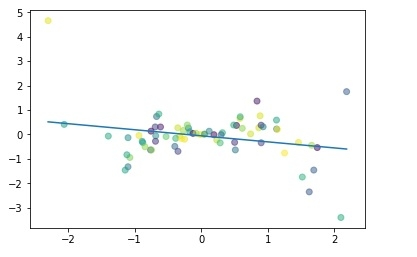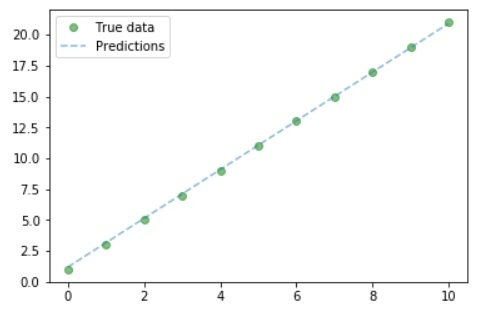# Linear Regression using PyTorch?

### Simple Linear Regression Basics

• Allows us to understand relationship between two continuous variable.

• Example −

• x = independent variable

• weight

• y = dependent variable

• height

• y = αx + β

Let's understand simple linear regression through a program −

#Simple linear regression

import numpy as np
import matplotlib.pyplot as plt

np.random.seed(1)
n = 70
x = np.random.randn(n)
y = x * np.random.randn(n)

colors = np.random.rand(n)
plt.plot(np.unique(x), np.poly1d(np.polyfit(x, y, 1))(np.unique(x)))

plt.scatter(x, y, c = colors, alpha = 0.5)
plt.show()

## Output### Purpose of Linear Regression:

• to Minimize the distance between the points and the line (y = αx + β)

• Coefficient: α

• Intercept/Bias: β

### Building a Linear Regression Model with PyTorch

Let's suppose our coefficient (α) is 2 and intercept (β) is 1 then our equation will become −

y = 2x +1 #Linear model

### Building the Dataset

x_values = [i for i in range(11)]
x_values

### Output

[0, 1, 2, 3, 4, 5, 6, 7, 8, 9, 10]

#convert to numpy

x_train = np.array(x_values, dtype = np.float32)
x_train.shape

### Output

(11,)
#Important: 2D required
x_train = x_train.reshape(-1, 1)
x_train.shape

### Output

(11, 1)
y_values = [2*i + 1 for i in x_values]
y_values

### Output

[1, 3, 5, 7, 9, 11, 13, 15, 17, 19, 21]
#list iteration

y_values = []
for i in x_values:
result = 2*i +1
y_values.append(result)

y_values

### Output

[1, 3, 5, 7, 9, 11, 13, 15, 17, 19, 21]
y_train = np.array(y_values, dtype = np.float32)
y_train.shape

### Output

(11,)
#2D required
y_train = y_train.reshape(-1, 1)
y_train.shape

### Output

(11, 1)

### Building Model

#import libraries
import torch
import torch.nn as nn

#Create Model class
class LinearRegModel(nn.Module):
def __init__(self, input_size, output_size):
super(LinearRegModel, self).__init__()
self.linear = nn.Linear(input_dim, output_dim)

def forward(self, x):
out = self.linear(x)
return out

input_dim = 1
output_dim = 1

model = LinearRegModel(input_dim, output_dim)

criterion = nn.MSELoss()

learning_rate = 0.01
optimizer = torch.optim.SGD(model.parameters(), lr = learning_rate)

epochs = 100
for epoch in range(epochs):
epoch += 1
#convert numpy array to torch variable
inputs = Variable(torch.from_numpy(x_train))
labels = Variable(torch.from_numpy(y_train))

#Forward to get output
outputs = model.forward(inputs)

#Calculate Loss
loss = criterion(outputs, labels)

loss.backward()

#Updating parameters
optimizer.step()

print('epoch {}, loss {}'.format(epoch, loss.data))

### Output

epoch 1, loss 276.7417907714844
epoch 2, loss 22.601360321044922
epoch 3, loss 1.8716105222702026
epoch 4, loss 0.18043726682662964
epoch 5, loss 0.04218350350856781
epoch 6, loss 0.03060017339885235
epoch 7, loss 0.02935197949409485
epoch 8, loss 0.02895027957856655
epoch 9, loss 0.028620922937989235
epoch 10, loss 0.02830091118812561
......
......
epoch 94, loss 0.011018744669854641
epoch 95, loss 0.010895680636167526
epoch 96, loss 0.010774039663374424
epoch 97, loss 0.010653747245669365
epoch 98, loss 0.010534750297665596
epoch 99, loss 0.010417098179459572
epoch 100, loss 0.010300817899405956

So we can the loss is reduced considerably from epoch 1 to epoch 100.

### Plot the graph

#Purely inference
predicted = model(Variable(torch.from_numpy(x_train))).data.numpy()
predicted
y_train

#Plot Graph

#Clear figure
plt.clf()

#Get predictions
predicted = model(Variable(torch.from_numpy(x_train))).data.numpy()

#Plot true data
plt.plot(x_train, y_train, 'go', label ='True data', alpha = 0.5)

#Plot predictions
plt.plot(x_train, predicted, '--', label='Predictions', alpha = 0.5)

#Legend and Plot
plt.legend(loc = 'best')
plt.show()

### OutputSo we can from the graph- our true and predicted value almost similar.

Updated on: 30-Jul-2019

306 Views